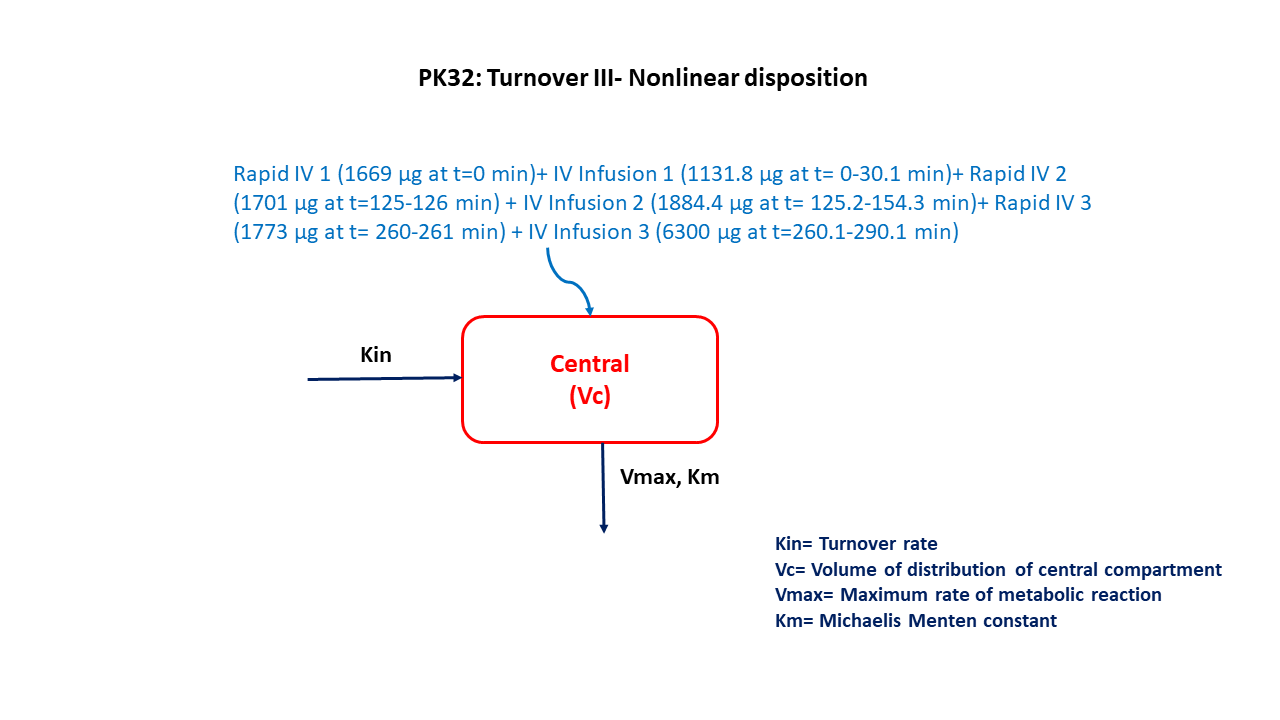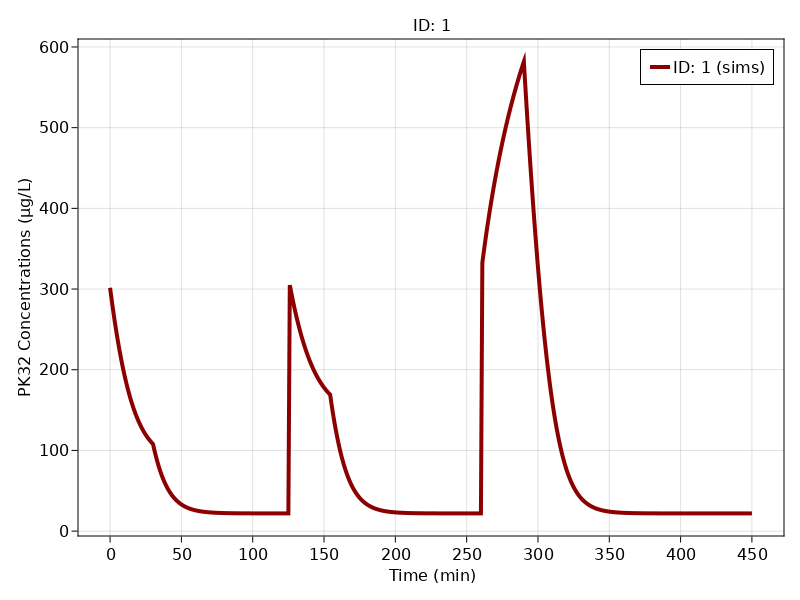# Exercise PK32 - Turnover III Nonlinear disposition

### Background

• Structural model - One compartment with zero order input and non linear elimination

• Route of administration - IV infusion

• Dosage Regimen - Multiple intravenous infusions (Three sets of Rapid iv infusion followed by slow iv infusion)

• Number of Subjects - 1### Learning Outcomes

We will learn to simulate data for Multiple infusions of an endogenous compound with non-linear disposition

### Objectives

• To build a one compartment model for an endogenous compound with non-linear disposition

• To use final parameter estimates and design a multiple infusion dosage regimen

• To simulate and plot a single subject with predefined time points.

### Libraries

Call the "necessary" libraries to get started.

using Random
using Pumas
using PumasUtilities
using CairoMakie


### Model

One compartment model for an endogenous compound with non-linear disposition

pk_32        = @model begin
desc     = "Non-linear Elimination Model"
timeu    = u"minute"
end

@param begin
"Volume of Central Compartment (L)"
tvvc     ∈ RealDomain(lower=0)
"Maximum metabolic capacity (μg/min)"
tvvmax   ∈ RealDomain(lower=0)
"Michaelis-Menten constant (μg/L)"
tvkm     ∈ RealDomain(lower=0)
"Rate of synthesis (μg/min)"
tvkin    ∈ RealDomain(lower=0)
Ω        ∈ PDiagDomain(4)
"Proportional RUV"
σ²_prop  ∈ RealDomain(lower=0)
end

@random begin
η        ~ MvNormal(Ω)
end

@pre begin
Vc       = tvvc*exp(η)
Vmax     = tvvmax*exp(η)
Km       = tvkm*exp(η)
Kin      = tvkin*exp(η)
#CL      = Vmax/(Km+(Central/Vc))
end

@init begin
Central  = Kin/((Vmax/Km)/Vc)
end

@dynamics begin
Central' = Kin - (Vmax/(Km+Central/Vc))*(Central/Vc)
end

@derived begin
cp       = @. Central/Vc
"""
Observed Concentration (ug/L)
"""
dv       ~ @. Normal(cp, sqrt(cp^2*σ²_prop))
end
end

PumasModel
Parameters: tvvc, tvvmax, tvkm, tvkin, Ω, σ²_prop
Random effects: η
Covariates:
Dynamical variables: Central
Derived: cp, dv
Observed: cp, dv


### Parameters

The parameters are as given below. tv represents the typical value for parameters.

• $Vc$ - Volume of Central Compartment (L)

• $Vmax$- Maximum metabolic capacity (μg/min)

• $Km$ - Michaelis- menten constant (μg/L)

• $Kin$ - Rate of synthesis,Turnover rate (μg/min)

• $Ω$ - Between Subject Variability

• $σ$ - Residual error

param = (tvvc    = 5.94952,
tvvmax  = 361.502,
tvkm    = 507.873 ,
tvkin   = 14.9684,
Ω       = Diagonal([0.00,0.00,0.00,0.00]),
σ²_prop = 0.05)

(tvvc = 5.94952, tvvmax = 361.502, tvkm = 507.873, tvkin = 14.9684, Ω = [0.
0 0.0 0.0 0.0; 0.0 0.0 0.0 0.0; 0.0 0.0 0.0 0.0; 0.0 0.0 0.0 0.0], σ²_prop
= 0.05)


### Dosage Regimen

DosageRegimen (DR) = Three sets of rapid intravenous infusion followed by slow intravenous infusion as followed

• IV bolus of 1669 μg (Time=0 min) followed by IV infusion of 1131.8 μg (Time= 0-30.1 min)

• IV infusion of 1701 μg (Time= 0-30.1 min) followed by IV infusion of 1884.4 μg (Time= 125.2-154.3 min)

• IV infusion of 1773 μg (Time= 260-261 min) followed by IV infusion of 6300 μg (Time= 260.1-290.1 min)

IVinfRapid = DosageRegimen([1669,1701,1733], time = [0,125,260], cmt = [1,1,1], duration = [0,1,1])
IVinfSlow  = DosageRegimen([1131.8,1884.4,6300], time = [0,125.2,260.1], cmt = [1,1,1], duration = [30.1,29.1,30])
DR         = DosageRegimen(IVinfRapid,IVinfSlow)
sub1       = Subject(id = 1, events = DR)

Subject
ID: 1
Events: 11


### Simulation

To simulate plasma concentration for single subject with the specific observation time points for a given dosage regimen 'DR'

Random.seed!(123)
sim  =  simobs(pk_32, sub1, param, obstimes=0:0.01:450)


### Visualization

f, a, p = sim_plot(pk_32, [sim],
observations = :cp,
color = :redsblues,
linewidth = 4,
axis = (xlabel = "Time (min)",
ylabel = "PK32 Concentrations (μg/L)",
xticks = 0:50:450, ))
axislegend(a)
f